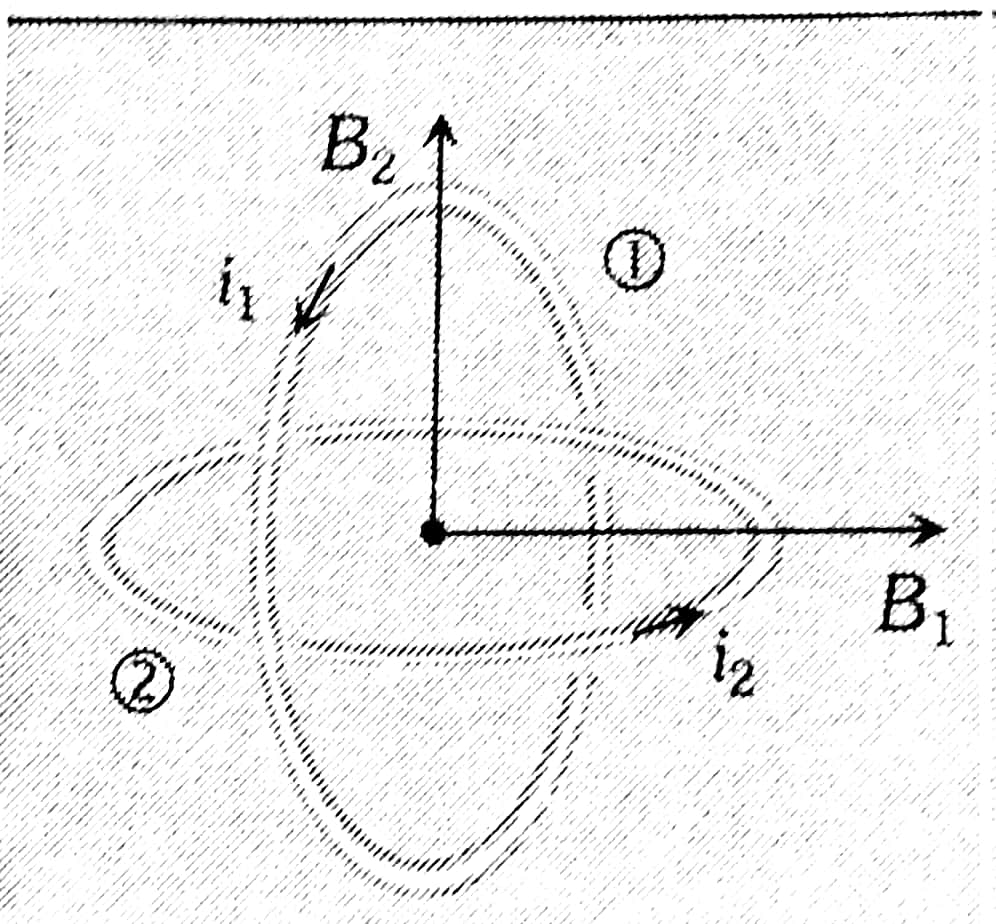#### Two concentric coils each of radius equal tocm are placed at right angles to each other. 3 ampere and 4 ampere are the currents flowing in each coil respectively. The magnetic induction in weber/m2  at the center of the coils will beOption 1)Option 2)Option 3)Option 4)As we learnt in

Concentric loops but their planes are perpendicular to each other -- whereinMagnetic induction at centre of one coilorOption 1)Correct option

Option 2)Incorrect option

Option 3)Incorrect option

Option 4)Incorrect option

#### Plabita